Electron. J. Diff. Equ., Vol. 2014 (2014), No. 13, pp. 1-6.

### Bifurcation from infinity and nodal solutions of quasilinear elliptic differential equations Bian-Xia Yang

Abstract:
In this article, we establish a unilateral global bifurcation theorem from infinity for a class of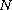-dimensional p-Laplacian problems. As an application, we study the global behavior of the components of nodal solutions of the problem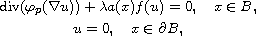where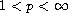,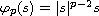,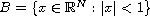, and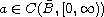is radially symmetric with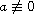on any subset of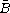,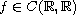and there exist two constants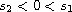, such that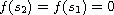, and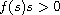for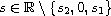. Moreover, we give intervals for the parameter, where the problem has multiple nodal solutions if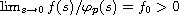and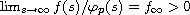. We use topological methods and nonlinear analysis techniques to prove our main results.

Submitted November 29, 2013. Published January 8, 2014.
Math Subject Classifications: 35P30，35B32.
Key Words: p-Laplacian; bifurcation; nodal solutions.

Show me the PDF file (188 KB), TEX file, and other files for this article.Bian-Xia Yang School of Mathematics and Statistics Lanzhou University Lanzhou, Gansu 730000, China email: yanglina7765309@163.com